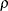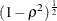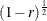POWER Statement

POWER <options> ;

The POWER statement performs power and sample size analyses for the Type III test of each effect in the model defined by the MODEL statements and for the contrasts defined by all CONTRAST statements. The MODEL statement must appear before the POWER statement if the EFFECTS option is used in the POWER statement.

Summary of Options

Table 44.5 summarizes the options available in the POWER statement.

Table 44.5: POWER Statement Options

Option

Description

Specifies the level of significance of each test

Specifies multiple correlation () between covariates and response

Specifies the location of the Dependent column in the output

Specifies the model effects

Specifies additional degrees of freedom due to covariates

Enables fractional input and output for sample sizes

Specifies the sample size

Controls ordering in output

Specifies Power

Specifies proportional variance reduction (r) due to covariates

Specifies error standard deviation

Table 44.6 summarizes the valid result parameters.

Table 44.6: Summary of Result Parameters in the POWER Statement

Solve for

Syntax

Power

POWER = .

Sample size

NTOTAL = .

Dictionary of Options

ALPHA=number-list

specifies the level of significance of each test. The default is 0.05, corresponding to the usual 0.05100% = 5% level of significance. Note that this is a test-wise significance level with the same value for all tests, not incorporating any corrections for multiple testing. See the section Specifying Value Lists in the POWER Statement for information about specifying the number-list.

CORRXY=number-list

specifies the multiple correlation () between all covariates and the response. The error standard deviation given by the STDDEV= option is consequently reduced by multiplying it by a factor of, provided that the number of covariates (as determined by the NCOVARIATES= option) is greater than zero. You cannot use the CORRXY= and the PROPVARREDUCTION= options simultaneously. See the section Specifying Value Lists in the POWER Statement for information about specifying the number-list.

DEPENDENT

specifies the location of the Dependent column in the output when the OUTPUTORDER=REVERSE option or OUTPUTORDER=SYNTAX option is used, according to its relative position in the POWER statement.

EFFECTS <= <( effect …effect ) >>

specifies the model effects to include in the power analysis. By default, or if the EFFECTS keyword is specified without the equal sign (=), all model effects are included. Specify EFFECTS=() to exclude all model effect tests from the power analysis. You can include main effects and interactions by using the effects notation of PROC GLM; see the section Specification of Effects in Chapter 42: The GLM Procedure, for further details. The MODEL statement must appear before the POWER statement if the EFFECTS option is used.

NCOVARIATES=number-list
NCOVARIATE=number-list
NCOVS=number-list
NCOV=number-list

specifies the number of additional degrees of freedom to accommodate covariate effects—both class and continuous—not listed in the MODEL statement. The error degrees of freedom are consequently reduced by the value of the NCOVARIATES= option, and the error standard deviation (whose unadjusted value is provided with the STDDEV= option) is reduced according to the value of the CORRXY= or PROPVARREDUCTION= option. See the section Specifying Value Lists in the POWER Statement for information about specifying the number-list.

NFRACTIONAL
NFRAC

enables fractional input and output for sample sizes. See the section Sample Size Adjustment Options for information about the ramifications of the presence (and absence) of the NFRACTIONAL option.

NTOTAL=number-list

specifies the sample size or requests a solution for the sample size with a missing value (NTOTAL=.). Values for the sample size must be no smaller than the model degrees of freedom (counting the covariates). See the section Specifying Value Lists in the POWER Statement for information about specifying the number-list.

OUTPUTORDER=INTERNAL | REVERSE | SYNTAX

controls how the input and default analysis parameters are ordered in the output. OUTPUTORDER=INTERNAL (the default) arranges the parameters in the output according to the following order of their corresponding options:

• weight variable (from the WEIGHT statement)

The OUTPUTORDER=SYNTAX option arranges the parameters in the output in the same order in which their corresponding options are specified in the POWER statement. The OUTPUTORDER=REVERSE option arranges the parameters in the output in the reverse of the order in which their corresponding options are specified in the POWER statement.

POWER=number-list

specifies the desired power of each test or requests a solution for the power with a missing value (POWER=.). The power is expressed as a probability (for example, 0.9) rather than a percentage. Note that this is a test-wise power with the same value for all tests, without any correction for multiple testing. See the section Specifying Value Lists in the POWER Statement for information about specifying the number-list.

PROPVARREDUCTION=number-list
PVRED=number-list

specifies the proportional reduction (r) in total R square incurred by the covariates—in other words, the amount of additional variation explained by the covariates. The error standard deviation given by the STDDEV= option is consequently reduced by multiplying it by a factor of, provided that the number of covariates (as determined by the NCOVARIATES= option) is greater than zero. You cannot use the PROPVARREDUCTION= and the CORRXY= options simultaneously. See the section Specifying Value Lists in the POWER Statement for information about specifying the number-list.

STDDEV=number-list

specifies the error standard deviation, or root MSE. If covariates are specified using the NCOVARIATES= option, then the STDDEV= option denotes the error standard deviation before accounting for these covariates. See the section Specifying Value Lists in the POWER Statement for information about specifying the number-list.

Restrictions on Option Combinations

For the relationship between covariates and response, specify either the multiple correlation (by using the CORRXY= option) or the proportional reduction in total R square (by using the PROPVARREDUCTION= option).